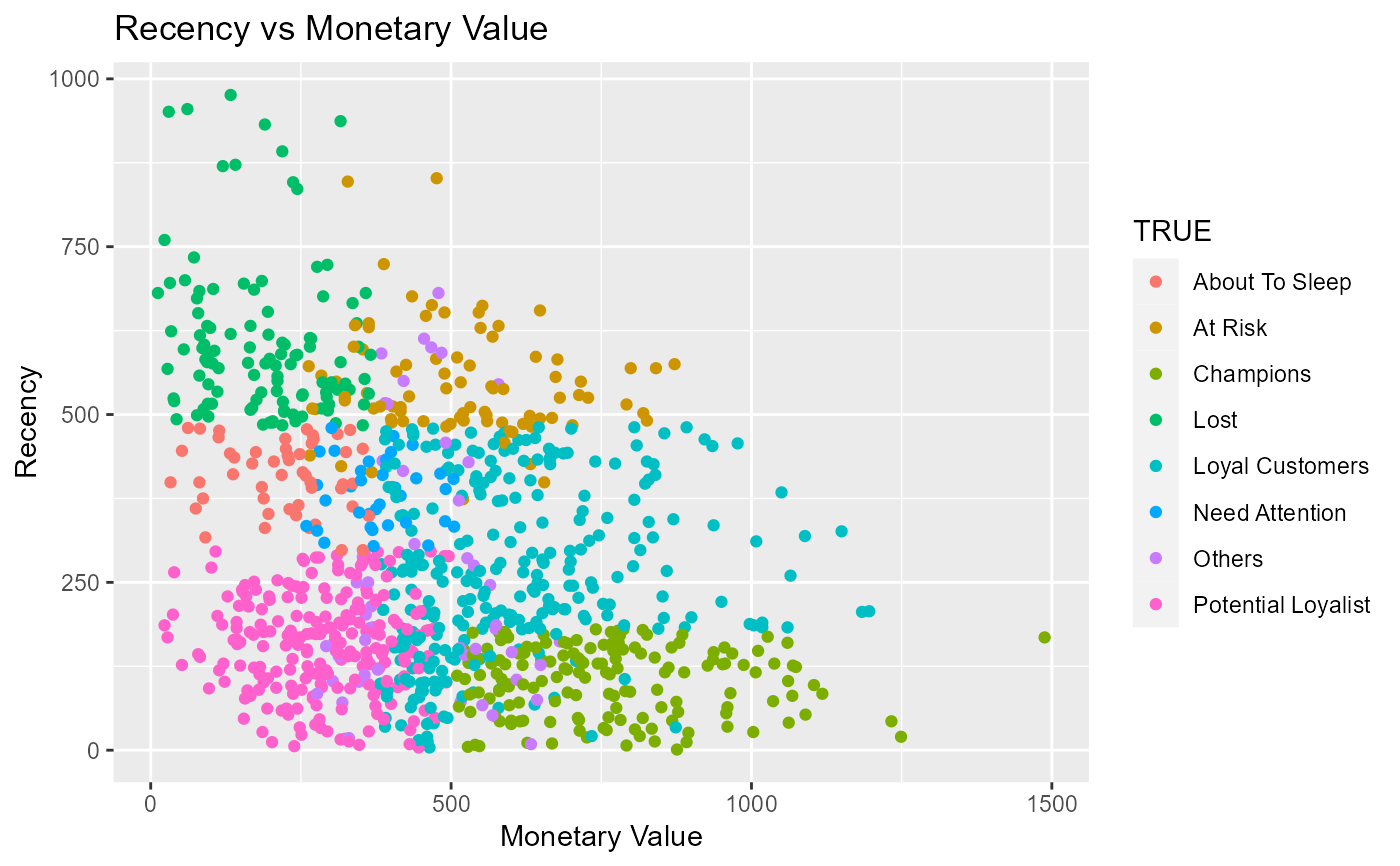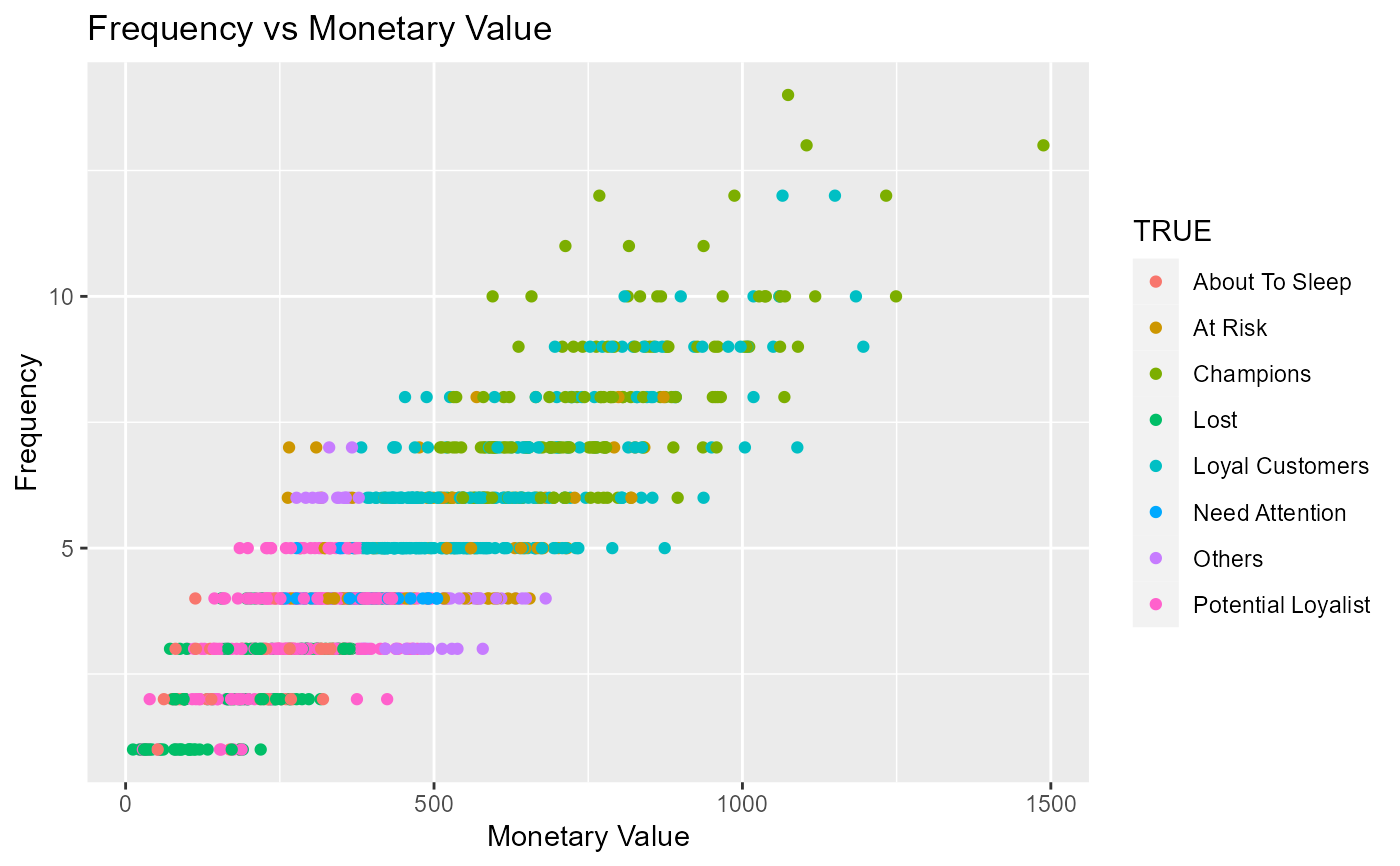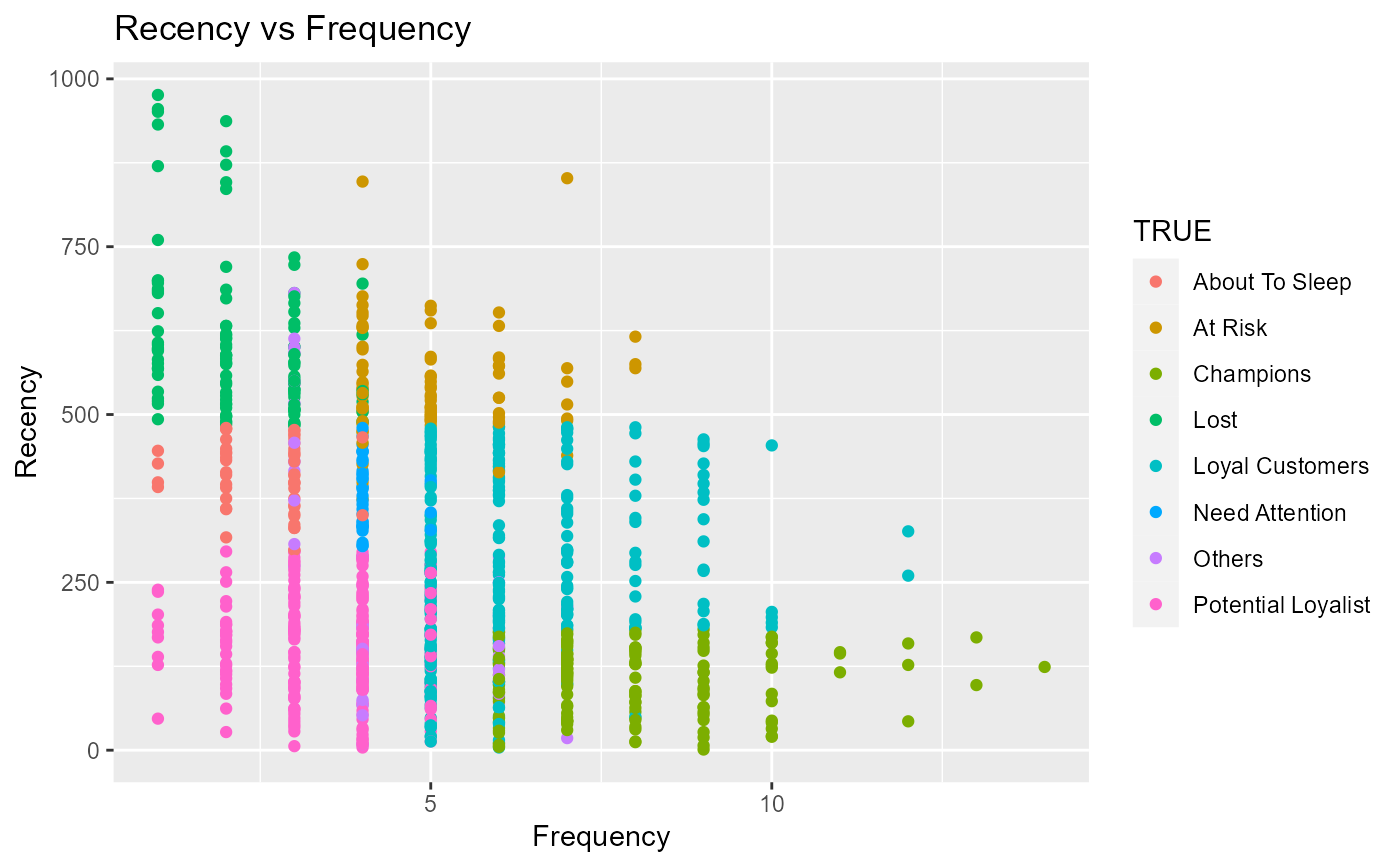Examine the relationship between recency, frequency and monetary values.

rfm_rm_plot(
rfm_table,
point_color = "blue",
xaxis_title = "Monetary",
yaxis_title = "Recency",
plot_title = "Recency vs Monetary",
print_plot = TRUE
)

rfm_fm_plot(
rfm_table,
point_color = "blue",
xaxis_title = "Monetary",
yaxis_title = "Frequency",
plot_title = "Frequency vs Monetary",
print_plot = TRUE
)

rfm_rf_plot(
rfm_table,
point_color = "blue",
xaxis_title = "Frequency",
yaxis_title = "Recency",
plot_title = "Recency vs Frequency",
print_plot = TRUE
)

## Arguments

rfm_table An object of class rfm_table. Color of the scatter points. X axis title. Y axis title. Title of the plot. logical; if TRUE, prints the plot else returns a plot object.

Scatter plot.

## Examples

# rfm table
analysis_date <- lubridate::as_date('2006-12-31')
rfm_result <- rfm_table_order(rfm_data_orders, customer_id, order_date,
revenue, analysis_date)

# monetary value vs recency
rfm_rm_plot(rfm_result)# frequency vs monetary value
rfm_fm_plot(rfm_result)# frequency vs recency
rfm_rf_plot(rfm_result)